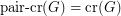# Pach, János

## Odd-cycle transversal in triangle-free graphs ★★

Author(s): Erdos; Faudree; Pach; Spencer

Conjecture   Ifis a simple triangle-free graph, then there is a set of at mostedges whose deletion destroys every odd cycle.

Keywords:

## Separators in string graphs ★★

Author(s): Fox; Pach; Tóth

Conjecture   Every string graph withedges has a separator of size.

Keywords: separator; string graphs

## Are different notions of the crossing number the same? ★★★

Author(s): Pach; Tóth

Problem   Does the following equality hold for every graph?The crossing numberof a graphis the minimum number of edge crossings in any drawing ofin the plane. In the pairwise crossing number, we minimize the number of pairs of edges that cross.

Keywords: crossing number; pair-crossing number